## Exercise 13.1 Page No: 208

1.Following are the car parking charges near a railway station upto:

4 hours   Rs.60

8 hours   Rs.100

12 hours   Rs.140

24 hours   Rs.180Check if the parking charges are in direct proportion to the parking time.

Solution:

Charges per hour:

C1 = 60/4 = Rs. 15

C2 = 100/8 = Rs. 12.50

C3 =  140/12 = Rs. 11.67

C4 = 180/24  = Rs.7.50

Here, the charges per hour are not same, i.e., C1 ≠ C2 ≠ C3 ≠ C4

Therefore, the parking charges are not in direct proportion to the parking time.

2. A mixture of paint is prepared by mixing 1 part of red pigments with 8 parts of base. In the following table, find the parts of base that need to be added.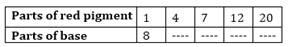Solution:

Let the ratio of parts of red pigment and parts of base be a/b .

Case 1: Here, a= 1, b= 8

a1/b1 = 1/8 = k (say)

Case 2: When  a= 4 , b= ?b2 = a2/k = 4/(1/8) = 4×8 = 32

Case 3: When  a3 = 7 , b= ?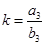b= a3/k = 7/(1/8) = 7×8 = 56

Case 4: When a= 12 , b=?b= a4/k = 12/(1/8) = 12×8 = 96

Case 5: When  a5 = 20 , b= ?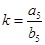b5 = a5/k = 20/(1/8) = 20×8 = 160

Combine results for all the cases, we have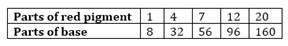3. In Question 2 above, if 1 part of a red pigment requires 75 mL of base, how much red pigment should we mix with 1800 mL of base?

Solution:

Let the parts of red pigment mix with 1800 mL base be  x.Since it is in direct proportion.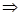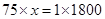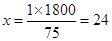Hence with base 1800 mL, 24 parts red pigment should be mixed.

4. A machine in a soft drink factory fills 840 bottles in six hours. How many bottles will it fill in five hours?Solution:

Let the number of bottles filled in five hours be x.

Here ratio of hours and bottles are in direct proportion.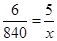6x = 5×840

x = 5×840/6 = 700

Hence machine will fill 700 bottles in five hours.

5. A photograph of a bacteria enlarged 50,000 times attains a length of 5 cm as shown in the diagram. What is the actual length of the bacteria? If the photograph is enlarged 20,000 times only, what would be its enlarged length?

Solution:

Let enlarged length of bacteria be  x .

Actual length of bacteria = 5/50000 = 1/10000  cm = 10-4  cmHere length and enlarged length of bacteria are in direct proportion.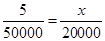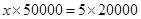x= 2cm

Hence the enlarged length of bacteria is 2 cm.

6. In a model of a ship, the mast is 9 cm high, while the mast of the actual ship is 12 m high. If the length if the ship is 28 m, how long is the model ship?Solution:

Let the length of model ship be  x .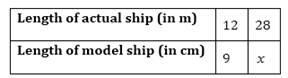Here length of mast and actual length of ship are in direct proportion.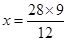x = 21 cm

Hence length of the model ship is 21 cm.

7. Suppose 2 kg of sugar contains 9×106 crystals. How many sugar crystals are there in

(i) 5 kg of sugar? (ii) 1.2 kg of sugar?

Solution:

(i) Let sugar crystals be  x.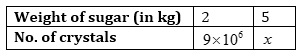Here, weight of sugar and number of crystals are in direct proportion.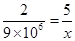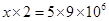=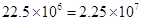Hence the number of sugar crystals is(ii)  Let sugar crystals be  x.

Here weight of sugar and number of crystals are in direct proportion.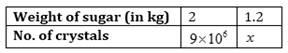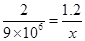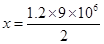=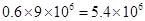Hence the number of sugar crystals is  5.4×106

8. Rashmi has a road map with a scale of 1 cm representing 18 km. She drives on a road for 72 km. What would be her distance covered in the map?

Solution:

Let distance covered in the map be  x.Here actual distance and distance covered in the map are in direct proportion.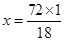x = 4 cm

Hence distance covered in the map is 4 cm.

9. A 5 m 60 cm high vertical pole casts a shadow 3 m 20 cm long. Find at the same time (i) the length of the shadow cast by another pole 10 m 50 cm high (ii) the height of a pole which casts a shadow 5 m long.

Solution:

Here height of the pole and length of the shadow are in direct proportion.

And 1 m = 100 cm

5 m 60 cm = 5×100+60 = 560 cm

3 m 20 cm = 3×100+20 = 320 cm

10 m 50 cm = 10×100+50 = 1050 cm

5 m = 5×100 = 500 cm

(i) Let the length of the shadow of another pole be  x.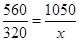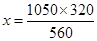x= 600 cm = 6m

Hence length of the shadow of another pole is 6 m.

(ii) Let the height of the pole be  x.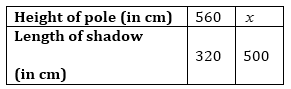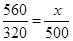= 875 cm = 8 m 75 cm

Hence height of the pole is 8 m 75 cm.

10. A loaded truck travels 14 km in 25 minutes. If the speed remains the same, how far can it travel in 5 hours?

Solution:

Let distance covered in 5 hours be x km.

1 hour = 60 minutes

Therefore, 5 hours = 5×60 = 300 minutesHere distance covered and time in direct proportion.25x = 300(14)x = 168

Therefore, a truck can travel 168 km in 5 hours.

## Exercise 13.2 Page No: 213

1. Which of the following are in inverse proportion?

(i) The number of workers on a job and the time to complete the job.

(ii) The time taken for a journey and the distance travelled in a uniform speed.

(iii) Area of cultivated land and the crop harvested.

(iv) The time taken for a fixed journey and the speed of the vehicle.

(v) The population of a country and the area of land per person.

Solution:

(i) The number of workers and the time to complete the job is in inverse proportion

because less workers will take more time to complete a work and more workers will take less time to complete the same work.

(ii) Time and distance covered in direct proportion.

(iii) It is a direct proportion because more are of cultivated land will yield more crops.

(iv) Time and speed are inverse proportion because if time is less, speed is more.

(v) It is a inverse proportion. If the population of a country increases, the area of land per person decreases.

2. In a Television game show, the prize money of Rs.1,00,000 is to be divided equally amongst the winners. Complete the following table and find whether the prize money given to an individual winner is directly or inversely proportional to the number of winners:Solution:

Here number of winners and prize money are in inverse proportion because winners are increasing, prize money is decreasing.

When the number of winners are 4, each winner will get =100000/4 = Rs. 25,000

When the number of winners are 5, each winner will get =100000/5 = Rs. 20,000

When the number of winners are 8, each winner will get =100000/8 = Rs. 12,500

When the number of winners are 10, each winner will get = 100000/10 = Rs. 10,000

When the number of winners are 20, each winner will get = 100000/20 = Rs. 5,000

3. Rehman is making a wheel using spokes. He wants to fix equal spokes in such a way that the angles between any pair of consecutive spokes are equal. Help him by completing the following table: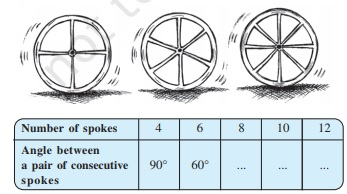(i) Are the number of spokes and the angles formed between the pairs of consecutive spokes in inverse proportion?

(ii) Calculate the angle between a pair of consecutive spokes on a wheel with 15 spokes.

(iii) How many spokes would be needed, if the angle between a pair of consecutive spokes is 40 degree ?

Solution:

Here the number of spokes are increasing and the angle between a pair of consecutive spokes is decreasing. So, it is a inverse proportion and angle at the centre of a circle is 360 degree .

When the number of spokes is 8, then angle between a pair of consecutive spokes = 360/8 = 45 degree

When the number of spokes is 10, then angle between a pair of consecutive spokes= 360/10= 36 degree.

When the number of spokes is 12, then angle between a pair of consecutive spokes= 360/12 = 30 degree.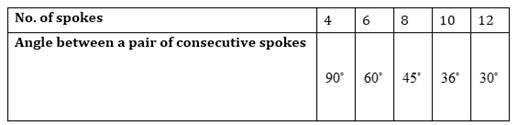(i) Yes, the number of spokes and the angles formed between a pair of consecutive spokes is in inverse proportion.

(ii) When the number of spokes is 15, then angle between a pair of consecutive spokes= 360/15= 24 degree.

(iii) The number of spokes would be needed = 360/40 = 9

4. If a box of sweets is divided among 24 children, they will get 5 sweets each. How many would each get, if the number of the children is reduced by 4?

Solution:

Each child gets = 5 sweets

24 children will get 24×5 = 120 sweets.

Total number of sweets = 120

If the number of children is reduced by 4, then children left = 24-4 = 20

Now each child will get sweets = 120/20 = 6 sweets

5. A farmer has enough food to feed 20 animals in his cattle for 6 days. How long would the food last if there were 10 more animals in his cattle?

Solution:

Let the number of days be x.

Total number of animals = 20+10 = 30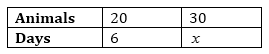Here the number of animals and the number of days are in inverse proportion.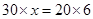x=4

Hence the food will last for four days.

6. A contractor estimates that 3 persons could rewire Jasminder’s house in 4 days. If, he uses 4 persons instead of three, how long should they take to complete the job?

Solution:

Let time taken to complete the job be x.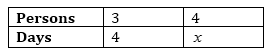Here the number of persons and the number of days are in inverse proportion.

¾ = x/4

3×4 = 4x

x = 3×4/4

x = 3

Hence, 4 persons will complete the job in 3 days.

7. A batch of bottles was packed in 25 boxes with 12 bottles in each box. If the same batch is packed using 20 bottles in each box, how many boxes would be filled?

Solution:

Let the number of boxes be x.Here the number of bottles and the number of boxes are in inverse proportion.

12/20 = x/25

12×25 = 20x

x = 12×25/20 = 15

Hence 15 boxes would be filled.

8. A factory requires 42 machines to produce a given number of articles in 63 days. How many machines would be required to produce the same number of articles in 54 days?

Solution:

Let the number of machines required be x.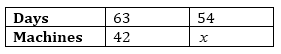Here the number of machines and the number of days are in inverse proportion.

63/54 = x/42

63×42 = 54x

x = 63×42/54

x= 49

Hence 49 machines would be required.

9. A car takes 2 hours to reach a destination by travelling at the speed of 60 km/hr. How long will it take when the car travels at the speed of 80 km/hr?

Solution :

Let the number of hours be x.Here the speed of car and time are in inverse proportion.

60/80 = x/2

60×2 = 80x

x = 60×2/8010. Two persons could fit new windows in a house in 3 days.

(i) One of the persons fell ill before the work started. How long would the job take now?

(ii) How many persons would be needed to fit the windows in one day?

Solution:

(i) Let the number of days be x.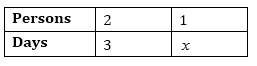Here the number of persons and the number of days are in inverse proportion.

2/1 = x/3

6 = x

Or x = 6 days

(ii) Let the number of persons be x.Here the number of persons and the number of days are in inverse proportion.

2/x = 1/3

6 = x

Or x = 6 persons

11. A school has 8 periods a day each of 45 minutes duration. How long would each period be, if the school has 9 periods a day, assuming the number of school hours to be the same?

Solution:

Let the duration of each period be x.Here the number of periods and the duration of periods are in inverse proportion.

8/9 = x/45

8×45 = 9x

x = 40

Hence, duration of each period would be 40 minutes.